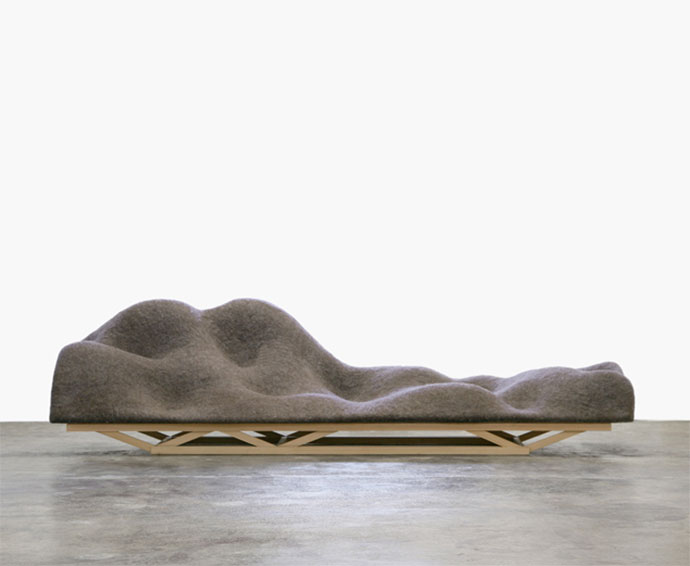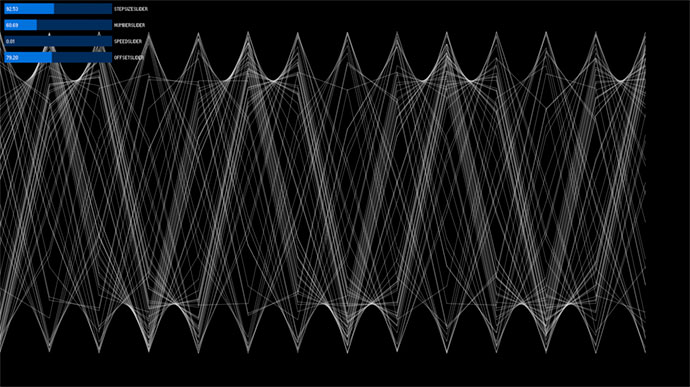# Tag 3 5.GUIBrainwave Sofa (Lucas Maassen) "The design of the Brain Wave Sofa is the result of a brain wave scan measured by means of an electro-encephalogram (EEG), using a set of electrodes connected to the head. A computer application for neuro-feedback displays the data as a 3D landscape image , in which the depth is the frequency of brain-activity in Hertz, the height is the strength of the signal and the length is the progression of time (duration). In this record and display mechanism, the role of the 'designer' is challenged; even by looking at the 3D visuals, you are directly influencing your design. In this case Maassen produced (created) the landscape by opening and closing his eyes while undergoing measurement of a specific wavelength known as the Alpha activity (8-12 Hertz). The Alpha activity is peculiar because when you close your eyes it strengthens, in contrast to other brain activity that declines. This is to prepare your brain for the large input of signals when you open your eyes."

Library controlP5

Beispiel 2-9``````
import controlP5.*;

ControlP5 cp5;

float x = 0;
float y = height/2;
float lastx = 0;
float lasty = 0;
float step = 0;
float stepSize = 1;
int number = 1;
float offsetAmount = 0;
float speed = 0.01;
float ang = 0;

void setup() {
size(1280,720);
fill(0,50);
stroke(255);
frameRate(60);
cp5 = new ControlP5(this);
.setPosition(10,10)
.setSize(200,20)
.setRange(1,200)
.setValue(1)
;
.setPosition(10,40)
.setSize(200,20)
.setRange(1,200)
.setValue(1)
;
.setPosition(10,70)
.setSize(200,20)
.setRange(0.01,1)
.setValue(0.01)
;
.setPosition(10,100)
.setSize(200,20)
.setRange(0,180)
.setValue(0)
;
}

void draw(){
background(0);
step += speed;
for (int count = 1; count <= number; count ++){
//float offset = random(offset);
float offset = randomGaussian()*offsetAmount;
for (int x=1; x < width; x+=stepSize){
y = (sin(ang)*sin(step)*height/2)+height/2;
if (x > lastx){
line(x, y, lastx, lasty);
}
lastx = x;
lasty = y;
}
}
}

void StepSizeSlider(float step){
stepSize = step;
}

void numberSlider(float num){
number = int(num);
}

void speedSlider(float sp){
speed = sp;
}

void offsetSlider(float off){
offsetAmount = off;
}

``````